# Assignment 2 - Linear Blend Skinning

Due Wednesday, 9/23 at 23:59:59. You must work individually.

## Goal

In this assignment, you will be creating a skinned animation of a character.

## Associated Labs

• Lab 0 (required): Setting Up Your Development Environment

## Starting point

Download the data/code for the assignment. The provided base code loads and displays a static mesh using indexed drawing. Run the code with the following arguments (modify as necessary depending on your IDE):

> ./A2 ../resources ../data

A character should appear in his “bind” pose. Create a README that contains the sentence, “The input data was downloaded from mixamo.com.”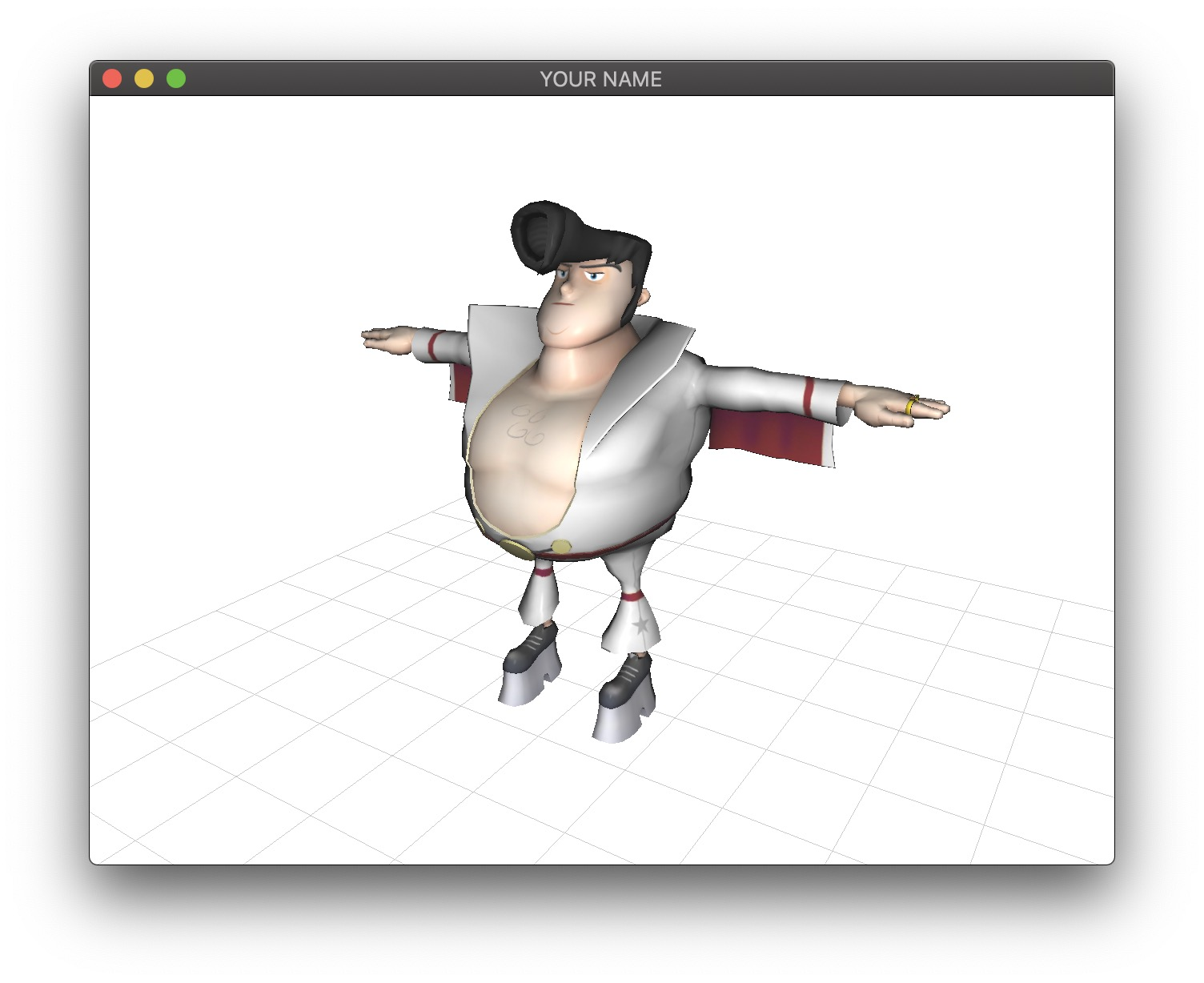Take a look at the files in the data/ folder. The contents are:

• input.txt: This is the first file that the program loads. It contains information about what other files to load.
• OBJ files: These are the mesh files. There are 4: body, brows, eyes, and mouth.
• Skin files: These contain the skinning information for the 4 mesh files.
• Skeleton files: These contain the frame-by-frame animation information for the bones.
• Two texture files: One file is for the body, and the other file is for the brows, eyes, and mouth.

## Skeleton

We are first going to load the skeleton file and display the bones as 3D axes.

The skeleton file (e.g., bigvegas_Walking_skel.txt) contains the animation sequence of the bones. The 4th line of the skeleton file contains the number of frames in the animation (27) and the number of bones (82). Each of the subsequent 27 lines defines the transforms of these 82 bones. In each line, there are $$82\times7=574$$ floats. Each block of 7 floats defines the quaternion and the position of a bone. In other words, each line is:

q0.x q0.y q0.z q0.w p0.x p0.y p0.z q1.x q1.y q1.z q1.w p1.x p1.y p1.z ...

Write a parser for this data, based on the example parser in loadDataInputFile() in main.cpp. (You can of course write your own parser from scratch if you prefer.) While parsing, be careful about the order of the elements for the quaternions. The data file stores x, y, z, w, not w, x, y, z. Once you parse the four scalars, you can create a glm::quat object by calling the constructor (assuming here that using namespace glm; has been called):

quat q(w, x, y, z);

Or, you can set the 4 elements individually:

quat q;
q.x = x;
q.y = y;
q.z = z;
q.w = w;

It is probably best to convert each 7-tuple (orientation quaternion and translation vector) into a single 4x4 rigid transformation matrix. The glm code to do this is:

mat4 E = mat4_cast(q);
E = vec4(p, 1.0f);

The very first data line in the skeleton file (line 5) defines the bind pose. You should save these transforms separately. The bind matrix for the zeroth (i.e., first) bone is (first 7 numbers of line 5):

1.0000         0         0         0
0    1.0000         0   96.3301
0         0    1.0000    9.8258
0         0         0    1.0000

The rest of the lines are the animation transforms. The zeroth animation matrix for the zeroth bone for bigvegas_Walking_skel.txt is (first 7 numbers of line 6):

 0.9956   -0.0481    0.0802    0.6576
0.0494    0.9987   -0.0153   87.5358
-0.0793    0.0192    0.9967    2.3914
0         0         0    1.0000

You can use the the to_string() method in glm to print out matrices:

#include <glm/gtx/string_cast.hpp>
...
glm::mat4 mat;
...
std::cout << glm::to_string(mat) << std::endl;

Note that this function prints the matrix column by column rather than row by row. In other words, it will display the transposed matrix. Remember that the translation factors should be in the right-most column, not the bottom row.

To debug the parsed data, draw the animation transformation matrices. You can press the ‘z’ key to toggle wireframe mode. For example, the bind pose should look like the following.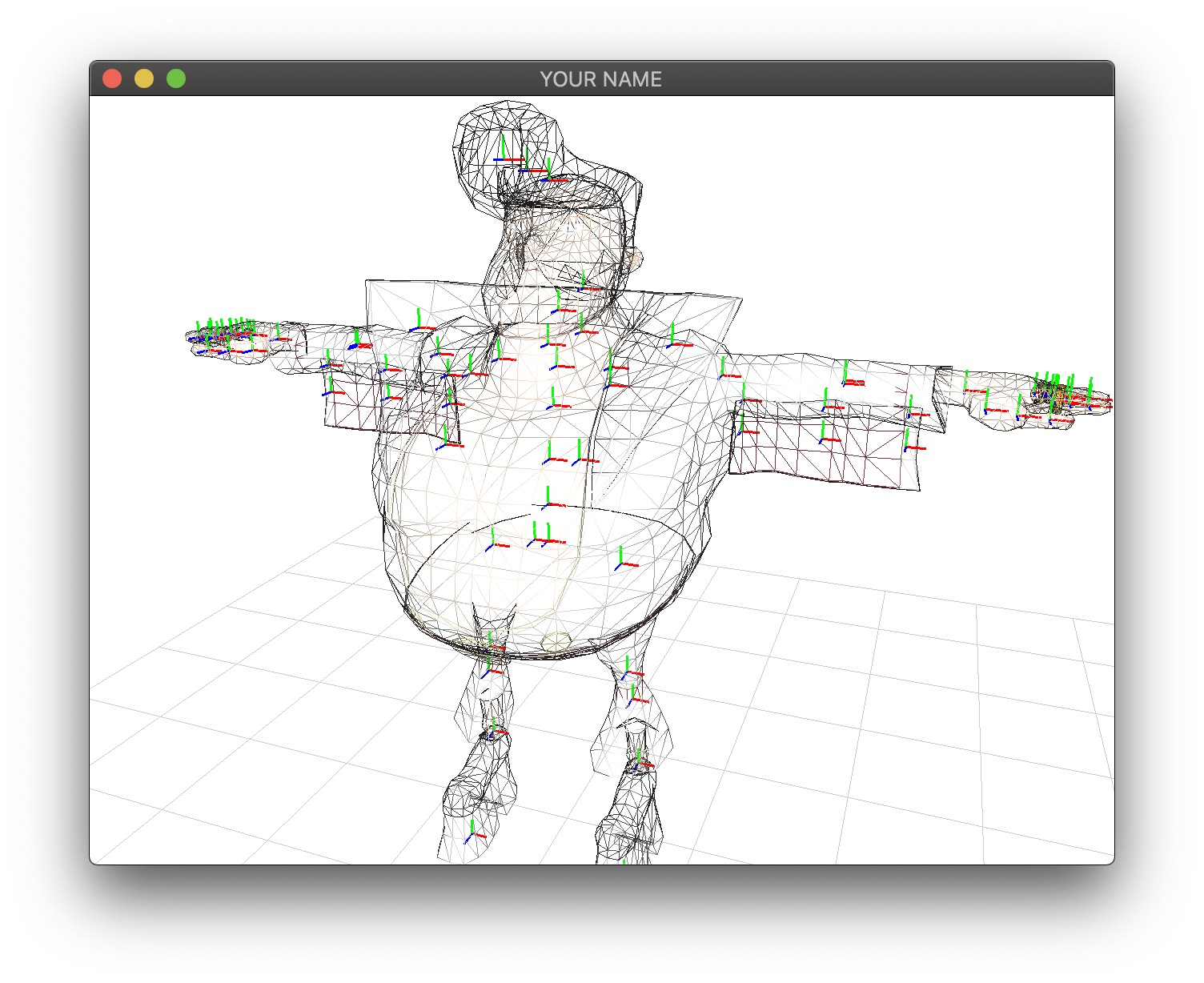Using the current “time” variable (obtained from glfwGetTime()), advance the animation so that you see a moving skeleton. The provided code already computes the local variable frame that you can use. You will need to set the frameCount variable to be the number of frames of the loaded skeleton file. Because we have not implemented skinning yet, the character will stay fixed – only the skeleton will move.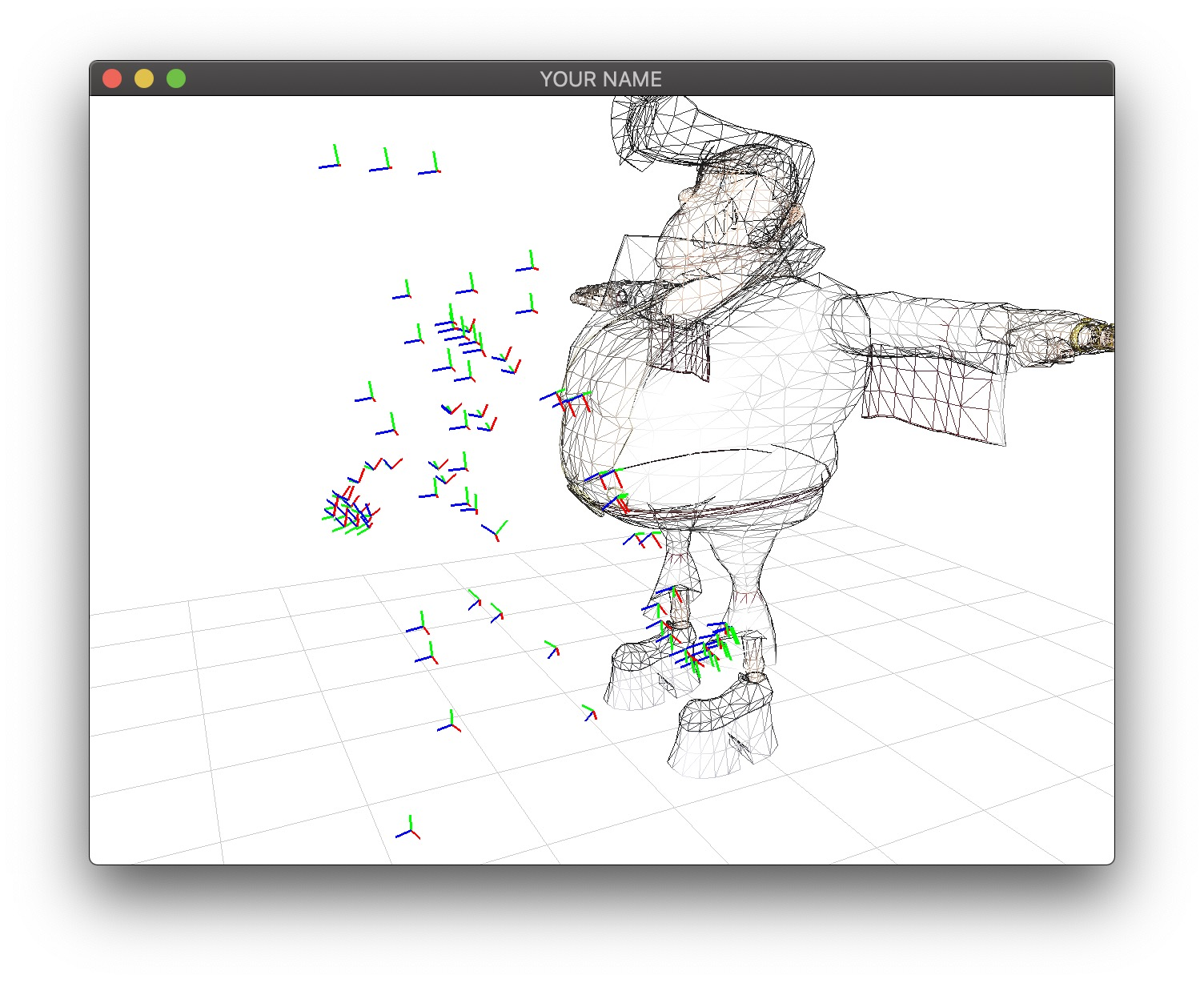## CPU Skinning

We are now ready to apply skinning to the vertices of the mesh.

### Attachment File

The attachment file contains the vertex skinning weights. Since there are 4 meshes (OBJ files), there are 4 attachment files. Take a look at bigvegas_BodyGeo_skin.txt. The 5th line contains the number of vertices (4583), the number of bones (82), and the maximum number of influences (9). The number of vertices should match the obj file, and the number of bones should match the skeleton file.

Each subsequent line of the attachment file defines the skinning weights of a vertex. The vertex ordering matches the obj file, so that the first vertex defined in the attachment file corresponds to the first vertex defined in the obj file. Each line starts with the number of influences for that vertex, followed by a sequence of (index, weight) pairs. For example, line 32 is:

3 18 0.015945 19 0.162761 20 0.821295 

This means that this particular vertex is influenced by 3 bones: 18, 19, and 20. The corresponding weights for these 3 bones are $$0.015945$$, $$0.162761$$, and $$0.821295$$. Note that the sum of these weights is $$0.015945 + 0.162761 + 0.821295 = 1.0$$. In fact, for each line, the sum of the weights is $$1.0$$.

Write a parser to load this data, again based on the example in loadDataInputFile(). The parsed data should be stored in the ShapeSkin class, so you should add some new member variables corresponding to bone indices (e.g., $$\{18, 19, 20\}$$) and skinning weights (e.g., $$\{0.015945, 0.162761, 0.821295\}$$). These new member variables (bone indices and skinning weights) should be vertex attributes just like vertex positions, normals, and texture coordinates. Also, just like how vertex positions, normals, and texture coordinates have the same size for each vertex (position has 3 floats, normal has 3 floats, texture coordinates has 2 floats for each vertex), bone indices and skinning weights should have the same size for each vertex. (For bigvegas_BodyGeo_skin.txt, each vertex should have 9 bone influences and 9 weights, padded with $$0$$ if a vertex is influenced by fewer than 9 bones (e.g., $$\{18, 19, 20, 0, 0, 0, 0, 0, 0\}$$ and $$\{0.015945, 0.162761, 0.821295, 0.0, 0.0, 0.0, 0.0, 0.0, 0.0\}$$).)

### CPU Skinning

Now that we have all the data loaded, we can implement basic skinning on the CPU. You’ll need to modify ShapeSkin.h, ShapeSkin.cpp, and main.cpp. You are free to add new methods, members, and classes as you see fit. The skinning equation for transforming the ith vertex position at the kth frame is:

$x_i(k) = \sum_{j \in J} w_{ij} M_j(k) (M_j(0))^{-1} x_i(0),$

where

• $$x_i(0)$$ is the initial position of the ith vertex, loaded from the obj file.
• $$J$$ is the set of bone influences for the ith vertex. For example, for line 32 above, $$J = \{18, 19, 20\}$$.
• $$M_j(0)$$ is the bind transformation matrix of the jth bone.
• $$M_j(k)$$ is the animation transformation matrix of the jth bone at kth frame.
• $$w_{ij}$$ is the skinning weight of the ith vertex for the jth bone. For example, for line 32 above, $$w_{ij} = \{ 0.015945, 0.162761, 0.821295 \}$$.
• $$x_i(k)$$ is the resulting skinned position of the ith vertex at kth frame.

You should compute this skinning equation in ShapeSkin::update() and update the position and normal buffers: posBuf and norBuf. In your code, it may be helpful to always use i for the vertex index, j for the bone index, and k for the frame index. The skinning equation for transforming the vertex normal is the same, assuming that $$M$$ is a rigid transform. Remember that the homogeneous coordinate of a position vector is $$1$$ and normal vector is $$0$$. Watch out to not modify the original positions and normals loaded from the obj file.

You need to transform each vertex using this equation before you draw the mesh. Note that it is more efficient to form the product $$M_j(k) M(0)^{-1}$$ for the 82 bones before looping over the 4583 vertices. Also, it is much better to invert the bind matrices once when you load them, since inverting the matrices every frame would be very wasteful.

### Sending the Data to the Shaders

To send the newly computed position and normal data to the shaders, you must move some lines from ShapeSkin::init() to ShapeSkin::update():

glBindBuffer(GL_ARRAY_BUFFER, posBufID);
glBufferData(GL_ARRAY_BUFFER, posBuf.size()*sizeof(float), &posBuf, GL_DYNAMIC_DRAW);

These lines tell the data in posBuf to be sent to the GPU. If these lines are in init() but not update(), then the position data do not get updated, and so the character would not move. This modification must be repeated for the normal data.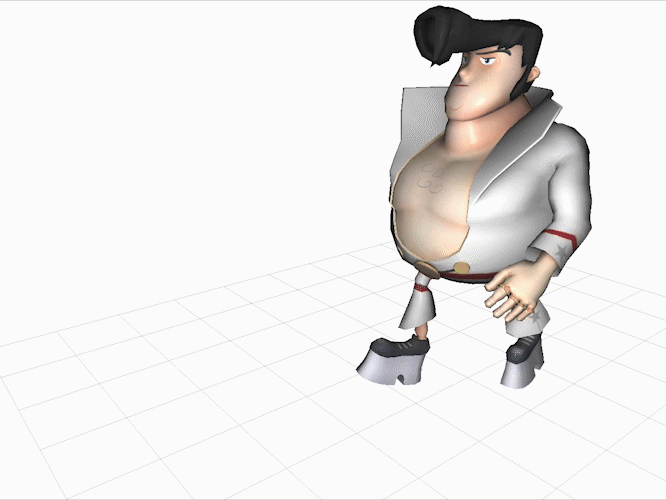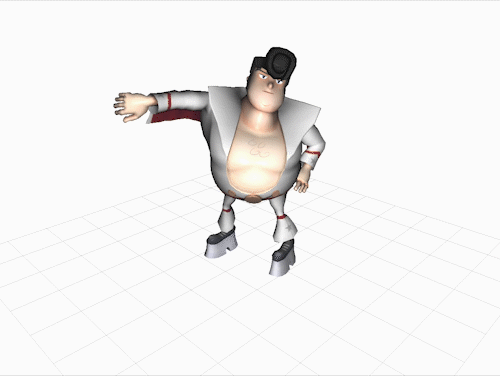## Texture Switching for Face

Take a look at MouthMAP.png (left image below).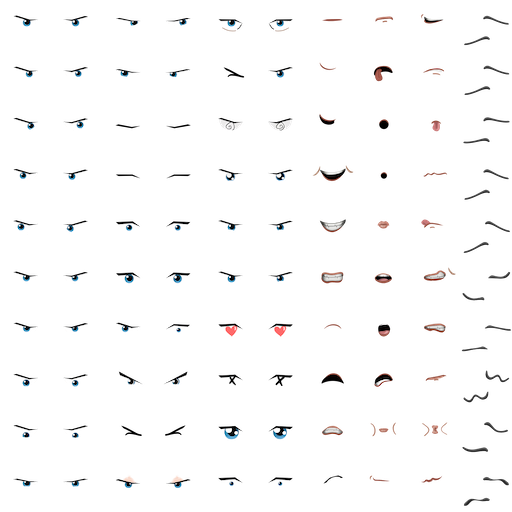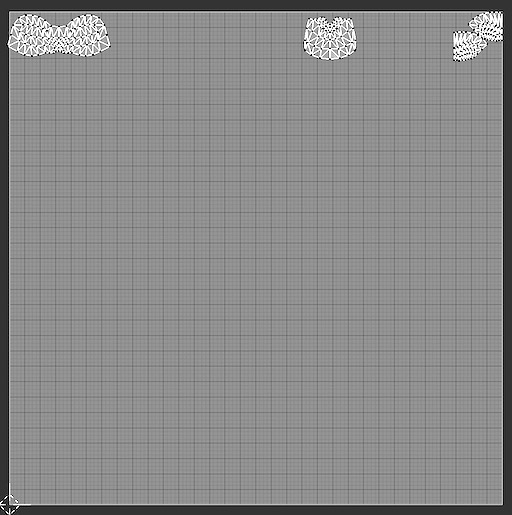Various styles of eyes, mouth, and the brows are defined within this single texture. The right figure shows the texture coordinates of the eyes, mouth, and brows meshes. To animate the face, we can translate the texture coordinates for these 3 meshes so that they cover different parts of the texture. To do so, use the texture matrix. Initially, the texture matrix is the identity matrix, so the textures at these default locations are used. By applying a translation to the texture matrix, we can make use of different parts of this texture file. Add some code in TextureMatrix::update() with the following key mappings:

• e Move the eye texture coordinates horizontally within the texture
• E Move the eye texture coordinates vertically within the texture
• m Move the mouth texture coordinates horizontally within the texture
• M Move the mouth texture coordinates vertically within the texture
• b Move the brow texture coordinates vertically within the texture

Make sure the texture coordinates wrap properly, so that if you press e three times, you get back to the original texture coordinates, and if you press E ten times, you also get back to the original texture coordinates.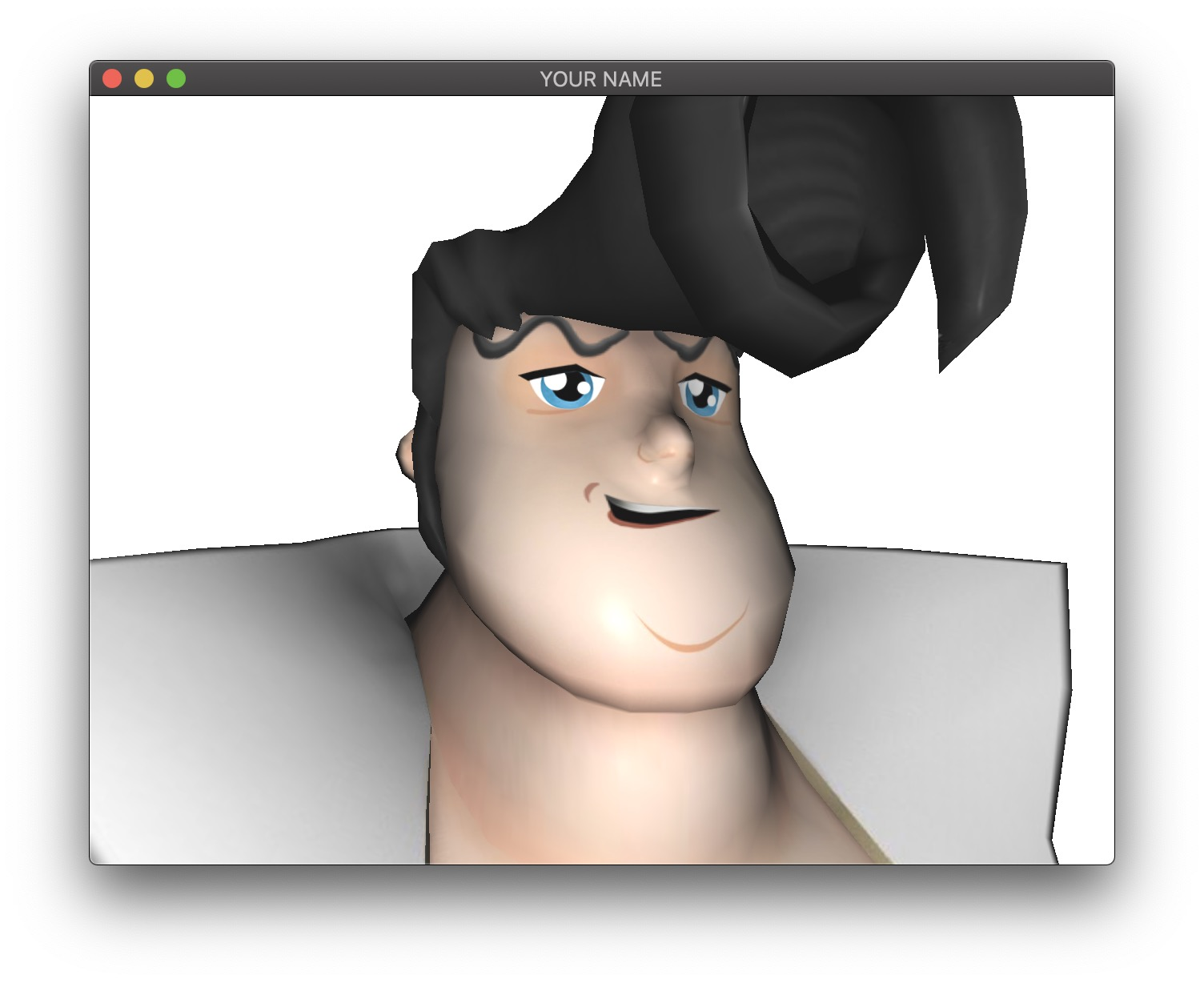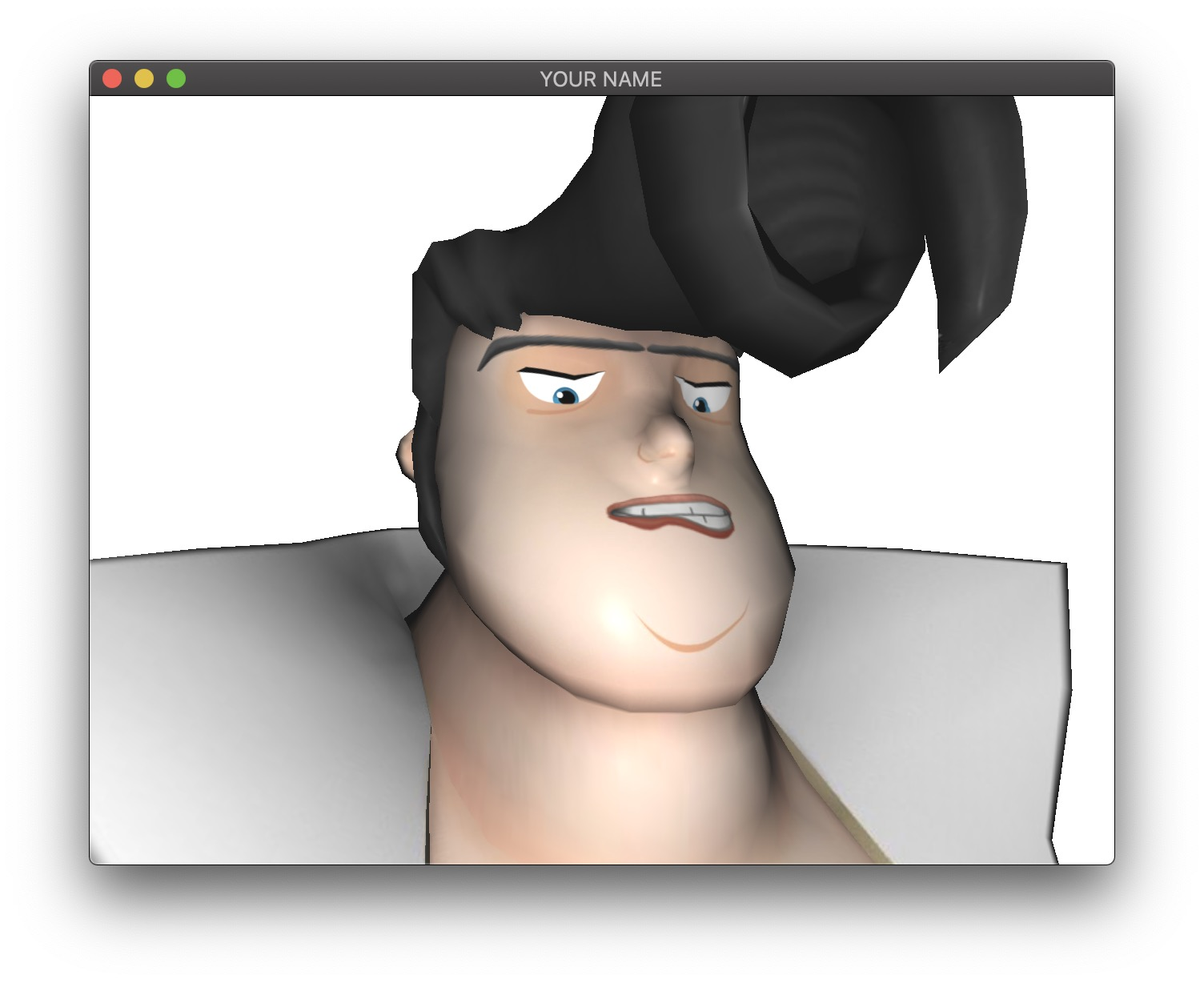## Bonus: GPU Skinning

Implement GPU skinning in the vertex shader. I recommend starting a new code base for the GPU version, since significant changes will be needed.

Let’s think about what information is needed in the vertex shader to compute the skinning calculations.

1. The initial “bind” positions and normals need to be passed in as vertex attributes. Since these do not change at run time, we can pass them in init() rather than in render().
2. The skinning weights and bone indices need to be passed in as vertex attributes. Again, these should be passed in in init().
3. The number of bone influences need to be passed in as a vertex attribute. This is the size of set $$J$$ in the skinning equation. Again, these should be passed in init().
4. The animation matrices need to be passed in as uniform data.

We will first deal with points 1-3 above: passing in the required vertex attributes.

In our dataset, the maximum number of bone influences per vertex is 9, so we will split up the skinning weights and bone indices into three vec4 attribute variables:

attribute vec4 weights0;
attribute vec4 weights1;
attribute vec4 weights2;
attribute vec4 bones0;
attribute vec4 bones1;
attribute vec4 bones2;
attribute float numInfl;

(You don’t need to use the variable names given above.) Since the maximum number of bone influences is 9, 3 vec4 variables are enough. The last elements in weights2 and bones2 will be padded with zeros. The last asttribute is the number of bone influences for this vertex. Although it is an integer, I’m using a float, which can be cast into an int with int(numInfl).

Even if you use 3 separate attribute variables in the GPU, you can still send the whole vertex attribute array as a single float array. As you have been doing for the other attributes, you should send the data to the GPU in the init() function rather than in the render() function:

glGenBuffers(1, &bufID);
glBindBuffer(GL_ARRAY_BUFFER, bufID);
glBufferData(GL_ARRAY_BUFFER, buf.size()*sizeof(float), &buf, GL_STATIC_DRAW);

Here, buf is a vector<float> that refers to the whole attribute array of all 12 weights (or bone indices). You can then tell OpenGL in the render() function about the stride length of each attribute.

glEnableVertexAttribArray(h_buf0);
glEnableVertexAttribArray(h_buf1);
glEnableVertexAttribArray(h_buf2);
glBindBuffer(GL_ARRAY_BUFFER, bufID);
unsigned stride = 12*sizeof(float);
glVertexAttribPointer(h_buf0, 4, GL_FLOAT, GL_FALSE, stride, (const void *)( 0*sizeof(float)));
glVertexAttribPointer(h_buf1, 4, GL_FLOAT, GL_FALSE, stride, (const void *)( 4*sizeof(float)));
glVertexAttribPointer(h_buf2, 4, GL_FLOAT, GL_FALSE, stride, (const void *)( 8*sizeof(float)));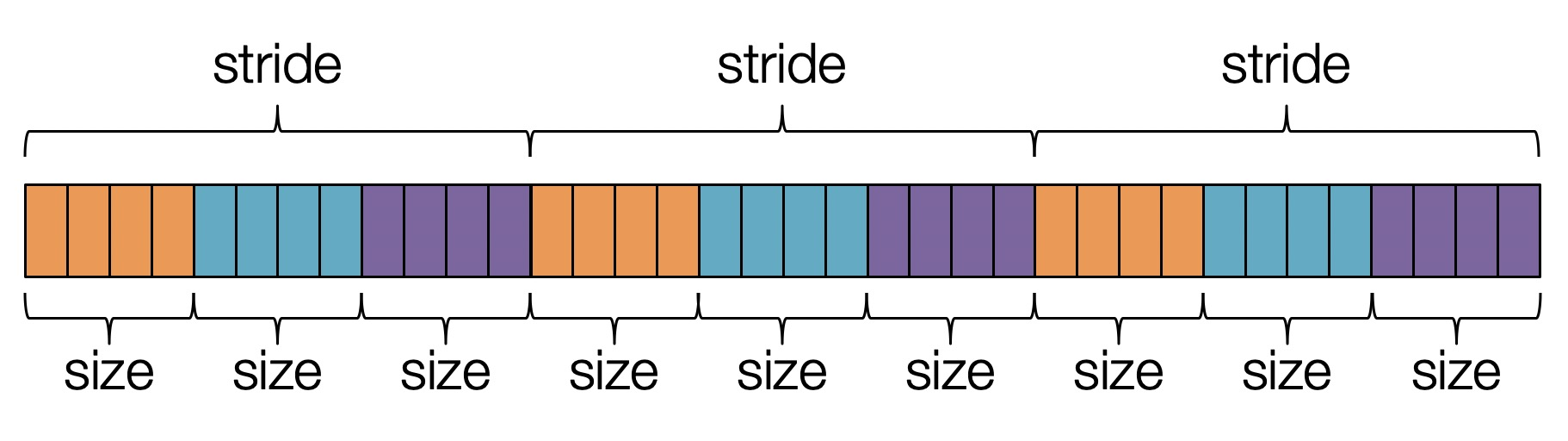This instructs OpenGL to take a single array for all 3 vec4 attributes but to treat each vec4 attribute as a separate attribute in the shader. The size is the number of elements in the attribute, which is 4. The stride is the skipping distance from one vertex to the next, which is 12. The last argument is the starting offset in the array.

You need to send in the bind and animation matrices as uniform variables. You can hardcode the number of matrices to be 82. (Note: there are 82 bones total, but the maximum number of bones influencing any single vertex is 9.) In the vertex shader, you should do something like:

uniform mat4 M;

To send in the matrix data from C++, you can create an array of matrices and pass in the address of the first element.

vector<mat4> M;
...
glUniformMatrix4fv(h_M, 82, GL_FALSE, glm::value_ptr(M));

There are 82 matrices, each of which is 16 floats. Be careful to not pass in the transpose. (The 3rd argument should be GL_FALSE in the function glUniformMatrix4fv().)

Don’t forget that you still need to apply the modelview and projection transforms to the skinned vertices.

## Bonus: More Data

You must complete CPU Skinning or GPU Skinning before attempting this bonus.

I created a C++ program to extract the skinning and animation data from an FBX file: fbx-extract. Using this code, try extracting more data from other FBX files you download from https://www.mixamo.com. This code was used to create the “BigVegas” data for this assignment, but it has not been tested otherwise. You may find bugs.

## Point breakdown

•  CPU skinning of positions.
•  CPU skinning of normals.
•  Efficiency: Animation must be smooth.
•  Texture switching
•  Coding style and general execution.
• [+25] GPU skinning.
• [+10] More data.

Total: 100 plus 35 bonus points.

## What to hand in

If you’re using Mac/Linux, make sure that your code compiles and runs by typing:

> mkdir build
> cd build
> cmake ..
> make
> ./A2 <SHADER DIR> <DATA DIR>

If you’re using Windows, make sure your code builds using the steps described in Lab 0.

• Put your name on the program window.
• Include an ASCII README that includes
• The copyright info for the mesh
• The highest stage you’ve completed
• Any bonus?
• Anything else of note
• Make sure you don’t get any compiler warnings.
• Remove unnecessary debug printouts.
• Remove unnecessary debug code, including commented out code.
• Do not hand in the data folder unless you created more data for bonus points.
• Do not hand in the executable, old save files (*.~), or object files (*.o). You should hand in the minimum set of files you need to compile plus the README file. Your “resources” directory should only contain your GLSL files.
• Create a single zip file of all the required files. The filename of this zip file should be USERNAME.zip (e.g., sueda.zip). The zip file should extract everything into a folder named USERNAME/ (e.g. sueda/).
• When you unzip this file, it should extract src/, resources/, CMakeLists.txt, and your README file to the current directory.
• Use the standard .zip format (not .gz, .7z, .rar, etc.).

Generated on Fri Sep 18 16:56:47 CDT 2020